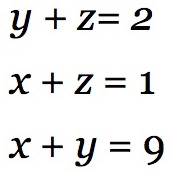Add up these 3 equations. What do you notice?

Find the values of x, y and z.

Now add up all 5 equations given below. What do you notice?

b + c + d + e = 4
a + c + d + e = 5
a + b + d + e = 1
a + b + c + e = 2
a + b + c + d = 0

Use the pattern you see in these equations to find the values of a, b, c , d and e.

Set your Twitter account name in your settings to use the TwitterBar Section.